# Current in simple DC circuits

What is electric current? We have attributed the forces between objects that are rubbed in particular ways to a property of matter known as charge. Most textbooks assert that the electric current that flows through the wires connected to a battery is charges in motion. How do we know this? Perhaps "current" is something else--another phenomenon.

This is a question that received a great deal of attention from Michael Faraday, one of the most famous scientists of the early nineteenth century. Faraday even studied the effects of electricity from animals such as electric eels. He concluded, "electricity, whatever may be its source, is identical in its nature."1

There is a demonstration set up in the center of the lab using the Whimshurst (German: Wimshurst) generator to show how the charge with which you are familiar can flow in simple wire circuits and can have a significant macroscopic effect!

In this unit, you are going to explore how charge originating in a battery or power supply flows in wires and bulbs. You will be asked to develop and explain some models that predict how the charge will flow. You will also be asked to devise ways to test your models using a device that measures the rate of flow of electrical charge through it, and displays it on the computer screen.

## Objectives

• To understand how a potential difference results in a current flow through a conductor.
• To learn to design and wire simple circuits using batteries, wires, light bulbs, and switches.
• To learn to use symbols to draw circuit diagrams.
• To understand the use of microcomputer-based probes for measuring current and voltage.
• To understand current flows at all points in simple circuits.

## Materials

• several flashlight bulbs (#14) in holders
• Connecting wires
• Two or three flashlight batteries (1.25 V. D cell)
• or an adjustable DC power supply.
• ULI with two current probes
• and two voltage probes and Logger Pro software

## Part Ia

### Procedure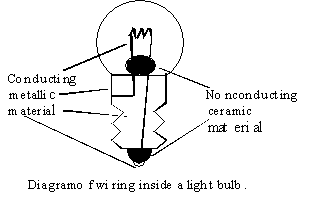Figure 1. Edison's incandescent bulb

1. Examine the bulb closely. Use a magnifying glass, if available. The figure above shows the parts of the bulb which are hidden from view.

2.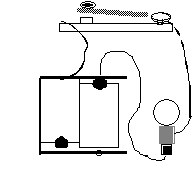Figure 2. Simple circuit with bulb.

3. Wire the circuit as in the figure, and test it. Note: For #14 light bulbs, do not use more than two batteries for a single bulb! Or for our bigger bulbs, no more than 6V on the DC power supply! Leave the switch closed so that the bulb remains on for 5 to 10 seconds. Feel the bulb. What did you feel? What is the result of the current flowing through the bulb?
4. What do you conclude about the path needed by the current to make the filament heat up and the bulb glow? Explain based on all the observations you have made so far?
5. Note that the Current Probe measures both the magnitude and direction of current flow.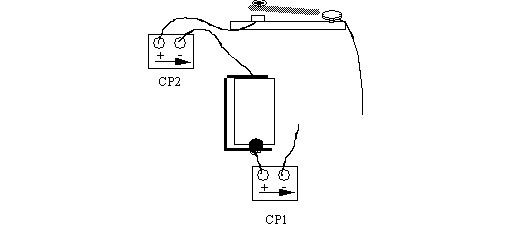Figure 4. Proper orientation

A current flowing in through the + terminal and out through the - terminal (in the direction of the arrow) will be displayed as a positive current. Thus, if the current measured by the probe in the figure is positive, you know that the current must flow from the + terminal of the battery, through the bulb, through the switch, and toward the - terminal of the battery.

For consistent results, you may use the power supply instead of a battery to supply the current. Your instructor or TA will show you the appropriate use of the power supply. Do not exceed 6 volts in any of the circuits in the lab.

You may measure current at up to two different places in the circuit simultaneously.

## Part Ib

1. Turn on the ULI and open the Logger Pro/ Open the file called Ohm's Law. (You can also open Current and Voltage Probes/ Two Current probes. Whichever you open, you will have to use the menubar setup/sensors to assign CH 1 & CH 2 as [Current & Voltage Probe - Current]. Assign both CH 3 and CH 4 as [Voltage (-10 V to 10V)]. Make sure the current and voltage are all off, then from the menubar "Zero all sensors" before connecting current probes.
2. Insert the current probes into the circuit as shown in the figure above. Be sure that the direction of the probes is the same for both and that the probes are set to measure current "flowing" from the positive battery terminal to the negative. If using power supply, do not turn up voltage past the tape mark (6V), otherwise you might burn out the light bulbs.
3. Be sure that Current Probes 1 and 2 are plugged into connectors 1 and 2 of the Current-Voltage box, and that the box is plugged into Ports CH 1 and 2 of the ULI.
4. To begin note in your lab notes the value of the current when the switch is open and when it is closed.
5. Try any other tests you need to decide on a current model. Sketch drawings of the circuits you used, showing where the probes were connected. Which model do you now think is the correct model for current flow? Why?

6. Note: As part of your lab reports, we will have you draw the circuits you have constructed. One could get tired quickly of drawing pictures of batteries, bulbs and switches. There are a series of symbols that have been created to represent circuits. Using these symbols will enable you to draw the nice neat square looking circuits that you see in physics textbooks. A few of the electric circuit symbols are shown below.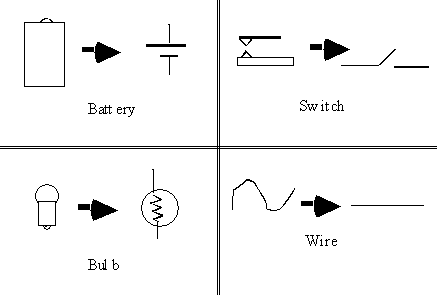Figure 5. Some common circuit symbols

Using these symbols, the standard circuits with a switch, bulb, wires, and battery can be represented as in the diagram on the right.Figure 6. A circuit sketch and corresponding diagram

## Part II Voltage and current

There are actually two important quantities to consider in describing the operation of electric circuits. One is current, and the other is potential difference, often referred to as voltage. As an introduction to our studies of more complex circuits, let's actually measure both current and voltage in a familiar circuit.Figure 7. Symbols and connections for Current Probe (left) and Voltage Probe (right)

This next figure shows the simple circuit with the battery and bulb with two Voltage Probes connected to measure the voltage across the battery and the voltage across the bulb. The circuit is drawn again symbolically on the right. Note that the word across is very descriptive of how the probes are connected to measure voltage.Figure 7b. Two Voltage Probes connected to measure the voltages across the battery and the bulb.

## Part III

1. Attach together black and red leads of each voltage probe and zero all sensors
2. Both voltage probes cannot be connected to circuit simultaneously unless they are connected with both having the correct polarity (i.e., 3 wrong ways, 1 right way to connect) otherwise a new current loop through the ULI is created. [It appears that the ULI puts a diode in each voltage probe that gives the probes a defined polarity.]
Connect the Voltage Probes to measure the voltage across the battery and the voltage across the bulb at the same time, connecting the black (-) lead of the probe to the red (+) lead of the battery and vice versa, doing the same for the bulb. If the bulb lights up with the switch open, you have reversed the probe polarity on the battery.
Record three voltage readings while closing and opening the switch several times, as before. Note any relationship between the voltages for each setting.
3. Now connect a Voltage and a Current Probe so that you are measuring the voltage across the battery and the current through the battery at the same time. See the next figure.

4.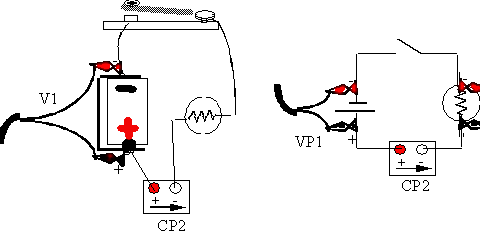Figure 8. Probes connected to measure the voltage across the battery and the current through it.

5. Record the reading for voltage and current as you open and close the switch. What relationship do you see here? Change the voltage probes to be over the bulb. Repeat the above readings.

### Analysis

What conclusion do you make concerning voltage and current through the battery and voltage and current through the bulb?

## Part IV Multiple bulbs

. What happens if more than one bulb is put in the circuit? There are actually two distinct ways of doing this. There are two arrangements, known as series and parallel that are shown in the diagrams below.Figure 9. Series connection

Note that the bulbs are connected in a series--the second bulb is connected to the positive terminal of the battery through the first bulb. In the same way, the first bulb is connected to the negative end of the battery through the second bulb.

Parallel connection: Note that both bulbs have a direct connection to the positive to the positive terminal of the battery and both are connected to the negative terminal directly through the switch.

1. Using the techniques and probes you used for the previous sections, measure the current and then the voltage in all the possible combinations. That is, there are three places to measure the current (after the power supply or battery, after the first bulb and after the second bulb) but only two current meters. Set the meters to measure the current after both bulbs, then after the battery or power supply and one bulb and then the battery and power supply and the other bulb. Repeat these measurements for the voltage as well. (So there are six possible combinations in all). Then repeat the whole set of measurements for the other configuration of the light bulbs (first series, then parallel).

## Analysis

Summarize your results in a table. State what conclusions you can about the current flow and voltage drop in series and parallel circuits.

## Extensions

1. Construct at least one of the devices described below. Sketch the circuit for each of your devices. Call over your TA when you have it working to check out your work!

A. Christmas Tree Lights: Suppose you want to light up your Christmas tree with three bulbs. Figure out a way to wire in all three bulbs so that the other two will still be lit if any one of the bulbs burns out. (Don't break the bulb! You can simulate failure by loosening a bulb in its socket.)

B. Lighting a Tunnel: The bulbs and switches must be arranged so that a person walking through a tunnel can turn on a lamp for a part of the tunnel and then turn on a second lamp in such a way that the first one turns off automatically.

C. Caller Indicator for the Deaf: A deaf person should be able to see, by looking at one or two bulbs, whether a visitor is at the front or back door of the house.
1Faraday,M. "Identity of Electricities Derived from Different Sources," in Experimental Researches in Electricity, Vol. I, Taylor and Francis, London. (Reprinted by Dover Publications, New York, 1965, p. 76).